# 相似多线外形之间特征曲线网的复制The Copy of Feature Curve Network among Similar Multiline Shapes

• 全文下载: PDF(4201KB)    PP.1782-1790   DOI: 10.12677/CSA.2018.811197
• 下载量: 228  浏览量: 375   国家自然科学基金支持

This paper is put forward to use smooth-deformation-mapping-fixed four steps to solve the problem of feature replication. First, it gives the Gauss smoothing algorithm of discrete curve. Then, it realizes the deformation of multi-line shape by the curve evolution algorithm. Next, it establishes a map-ping between two multi-line shapes to get the feature curve network. Finally, it fixes the feature curve network locally. The paper verifies the correctness of the algorithm on DateSet, which is a self- designed graphic and image processing software. This algorithm has a wide range of application and solves the problem of realizing the replication of the feature curve network rapidly and accurately.

1. 引言

2. 截面线点云的高斯光顺

$g\left(s,\sigma \right)=\frac{1}{\sigma \sqrt{2\text{π}}}{\text{e}}^{-\frac{{s}^{2}}{2{\sigma }^{2}}}$ ,

${g}_{w}=g\left(w,\sigma \right)=\frac{1}{\sigma \sqrt{2\text{π}}}{\text{e}}^{-\frac{{w}^{2}}{2{\sigma }^{2}}}$ , $w\in \left[-L,L\right]$ , $w\in Z$

3. 基于多线外形的特征曲线网变形

3.1. 基于ICP方法的刚体变换实现初始变形

${X}^{\prime }=HX$ , $H=\left[\begin{array}{cc}R& T\\ 0& 1\end{array}\right]$

$f\left(H\right)=f\left(R,T\right)=\underset{i=1}{\overset{N}{\sum }}‖{{Q}_{i}-R{P}_{i}-T‖}_{{}_{2}}^{2}$

$\mathrm{min}\left(f\left(H\right)\right)=\mathrm{min}\left(f\left(R,T\right)\right)=\mathrm{min}\left(\underset{i=1}{\overset{N}{\sum }}{‖{Q}_{i}-R{P}_{i}-T‖}^{2}\right)$ (1)

ICP算法能够保证得到的解局部最小，通过本节算法验证实例，得到了较好的初始配准结果，图4.6演示了通过ICP迭代进行初始变形的过程，其中图1(a)为三维变换后的两个多线外形，蓝色表示目标个体，红色表示经过ICP变形后的个体，图1(b)~(f)分别为迭代1，2，4，8，10次后的两个多线外形，可以看出，随着迭代次数的增大，两个多线外形越来越接近，表1给出了迭代过程中两个多线外形的平均误差。经过多次试验，本文将迭代终止条件设定为两个多线外形的平均距离小于5 mm，如表1所给个体需要迭代10次满足迭代终止条件。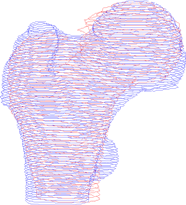(a)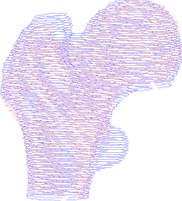(b)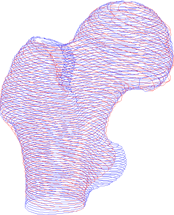(c)(d)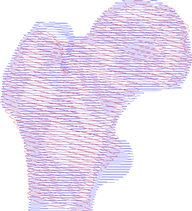(e)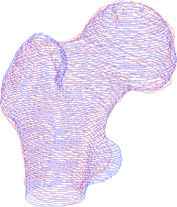(f)

Figure 1. Multi-line shapes for two hip femurs by initial deformation. (a) No iteration; (b) After 1 iteration step; (c) After 1 iteration steps; (d) After 4 iteration steps; (e) After 8 iteration steps; (f) After 10 iteration stepsTable 1. Mean error by ICP iterations

3.2. 收缩包围算法实现曲线演化

Step 1：对于 ${\Gamma }_{k}^{r}$ 上任一点 ${P}_{i,k}^{r}$，首先在 ${\Gamma }_{k}^{\infty }$ 上寻找最近点 ${P}_{j,k}^{\infty }$，设定距离阈值 ${d}_{\delta }$，通过判断 $|{P}_{i,k}^{r}{P}_{j,k}^{\infty }|\le {d}_{\delta }$ 来决定Step 2还是Step 3，若满足 $|{P}_{i,k}^{r}{P}_{j,k}^{\infty }|\le {d}_{\delta }$ 转Step 2，否则转Step 3；

Step 2：通过计算 ${P}_{i,k}^{r}{P}_{j,k}^{\infty }$ 在各自曲线上的法向 ${n}_{i,k}^{r}$${n}_{j,k}^{\infty }$，若 $\left({n}_{i,k}^{r},{n}_{j,k}^{\infty }\right)\ge 0$，说明该点是有效点，将该点传给 ${{\Gamma }^{\prime }}_{k}^{r}$

Step 3：L是连接 ${\Gamma }_{k}^{r}$ 上的所有点 ${P}_{i,k}^{r}$ 构成的多边形， ${P}_{k}^{\infty }$ 也是连接曲线 ${\Gamma }_{k}^{\infty }$ 上所有点构成的闭合的多边形。通过法向进行线段投影，将L上的点 ${P}_{i,k}^{r-1}$ 投影到 ${P}_{k}^{\infty }$，如果同时有好几个交点，选择到点 ${P}_{i,k}^{r}$ 距离最短的交点，设交点在所在曲线上的法向为 $n$，同时这个交点要满足 $\left(n,{n}_{i,k}^{r-1}\right)>0$ 由于像素点都是整数点，求出的交点可能不是整数，要把交点整数化，这样就能获得了 ${\Gamma }_{k}^{\infty }$ 上的点 ${P}_{j,k}^{\infty }$。如果此点 ${P}_{j,k}^{\infty }$ 满足Step 1中的条件，那么说明 ${P}_{j,k}^{\infty }$ 是有效的，同时把这点传递到 ${\Gamma }_{k}^{r}$，以便进行下一次的曲线投影；

Step 4：在演化过程中，检查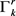上的点，如果两个点 ${{P}^{\prime }}_{i,k}^{r}$${{P}^{\prime }}_{i+1,k}^{r}$ 的索引号是相邻的，那么这两个点在相关联的图像中对应的像素不相邻的。就要使用线段离散化成像素，得到新的截面线 ${{\Gamma }^{\prime }}_{k}^{r}$，作为下一轮轮廓演化的初始轮廓；

Step 5：计算两曲线距离 ${N}_{\text{move}}$ (演化后的曲线与目标曲线的距离，通过两条曲线上点的距离和计算)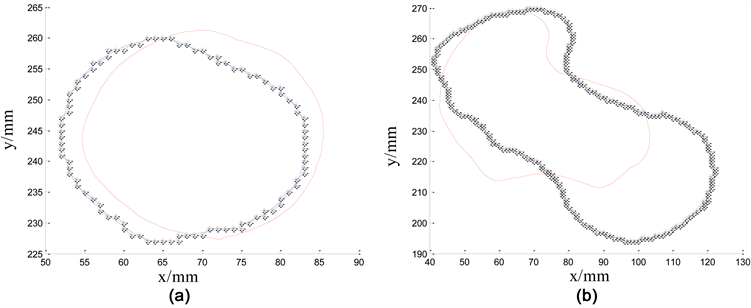Figure 2. Evolution of plane curves. (a) Curve evolution of example 1; (b) Curve evolution of example 2

4. 特征曲线网的映射和修正

4.1. 特征曲线网的映射

Step 1：对于两个多线外形，设截面线条数分别为 ${N}_{1}$${N}_{2}$，若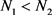，转Step 2；若 ${N}_{1}>{N}_{2}$ 转Step 3；

Step 2：将 ${N}_{2}$ 精简到与 ${N}_{1}$ 相同，从第一条到  条，隔行删除 $\left({N}_{2}-{N}_{1}\right)$ 条截面线，直到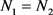.

Step 3：将 ${N}_{2}$ 插值得到与 ${N}_{1}$ 相同，从第一条到 ${N}_{2}$ 条，隔行插入 $\left({N}_{2}-{N}_{1}\right)$ 条截面线，直到 ${N}_{1}={N}_{2}$。插值的方法采用  $\lambda \in \left[0,1\right]$，其中 ${Q}_{i-1}$ 表示前一层截面线上的点集， ${Q}_{i-1}$ 表示后一层截面线上的点集， $\left(1-\lambda \right){Q}_{i-1}+\lambda {Q}_{i}$ 表示插值后得到的截面线上的点集.

Step 4：设两个多线外形统一截面线条数后共有 $N={N}_{1}$ 条，平均截面间隔为dis，设多线外形的高度(z轴方向长度)为 $D=|{z}_{N}-{z}_{1}|$ ( ${z}_{N}$ 表示截面线上最大z坐标，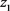表示截面线上最小z坐标)，则 $dis=\frac{D}{N}$，得到统一间隔dis后，分别对截面线调整z坐标，设第一层截面线的z坐标为 ${z}_{1}$，则第i层截面线的新的z坐标为 ${z}_{i}={z}_{1}+i×dis$ $\left(i=0,1,2,\cdots ,N-1\right)$ 经过上述两个步骤的调整，欲进行变形的多线外形A，B，具有相同的截面线条数和相同的截面间隔，于是可以建立一一映射关系。多线外形A的第i层截面线对应多线外形B的第i层截面线，因此我们可以利用曲线演化理论，将多线外形A的第i层截面线变形到多线外形B的第i层截面线。利用分层截面线变形来驱动整个多线外形变形其好处在于，截面线很好的保持了个体的形状，减少了曲面拟合产生的误差。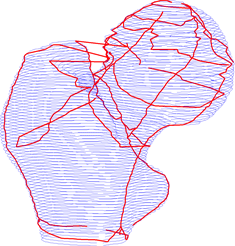(a)(b)

Figure 3. Feature curve networks for hip femurs. (a) Feature curve network for hip femur 1; (b) Feature curve network for hip femur 2

4.2. 映射后特征曲线网的修正

5. 小结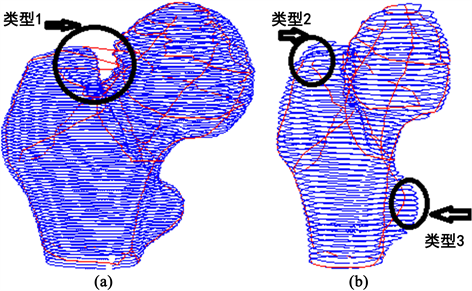Figure 4. Feature curve networks after mapping for hip femurs. (a) Type 1: redundancy; (b) Type 2: the missing; Type 3: non-feature points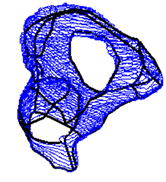(a)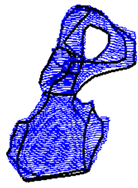(b)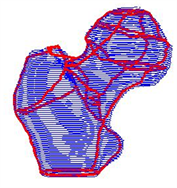(c)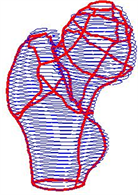(d)

Figure 5. Modified feature curve networks. (a) Hip femur 1; (b) Hip femur 2; (c) Hipbone 1; (d) Hipbone 2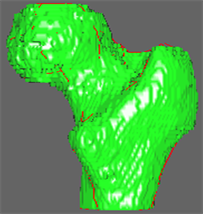(a)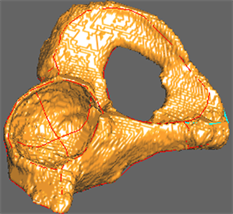(b)

Figure 6. Three-dimensional reconstruction of hip joints. (a) For hip femur; (b) For hipbone

NOTES

*通讯作者。

  Taubin, G. (1995) A Signal Processing Approach to Fair Surface Design. In: SIGGRAPH’95 Proceedings of the 22nd Annual Confer-ence on Computer Graphics and Interactive Techniques, ACM, New York, 351-358.  Desbrun, M., Meyer, M., Schroder, P., et al. (1999) Implicit Fairing of Irregular Meshes Using Diffusion and Curvature Flow. In: SIGGRAPH’99 Proceedings of the 26th An-nual Conference on Computer Graphics and Interactive Techniques, ACM Press/Addison-Wesley Publishing, New York, 317-324.  Pauly, M., Keiser, R., Kobbelt, L. and Gross, M. (2003) Shape Modeling with Point-Sampled Geometry. ACM Trans-actions on Graphics, 22, 641-650. https://doi.org/10.1145/882262.882319  Kara, L.B., D’Eramo, C.M. and Shimada, K.. (2006) Pen-Based Styling Design of 3D Geometry Using Concept Sketches and Template Models. SPM’06 Proceedings of the 2006 ACM Symposium on Solid and Physical Modeling, Cardiff, 6-8 June 2006, 149-160.  杨红娟, 周以齐, 陈成军. 基于多尺度分析的逆向工程截面线特征分割[J]. 计算机集成制造系统, 2007, 13(2): 375-380.  Mokhtarian, F. and Mackworth, A.K. (1992) A Theory of Multi-Scale, Curvature-Based Shape Representation for Planar Curves. IEEE Transactions on Pattern Analysis and Ma-chine Intelligence, 14, 789-805. https://doi.org/10.1109/34.149591  袁亮. 三维重建过程中的点云数据配准算法的研究[D]: [硕士学位论文]. 西安: 西安电子科技大学, 2010.  朱心雄, 等. 自由曲线曲面造型技术[M]. 北京: 科学出版社, 2008.  李晓志. 基于非接触式三维人体测量技术的人体数据处理与三维建模的研究[D]: [硕士学位论文]. 天津: 天津工业大学, 2007.  何姗姗, 刘浩, 唐月红. 基于股骨三维多线外形计算颈干角的方法[J]. 中国生物医学工程学报, 2013, 23(1): 109-113.